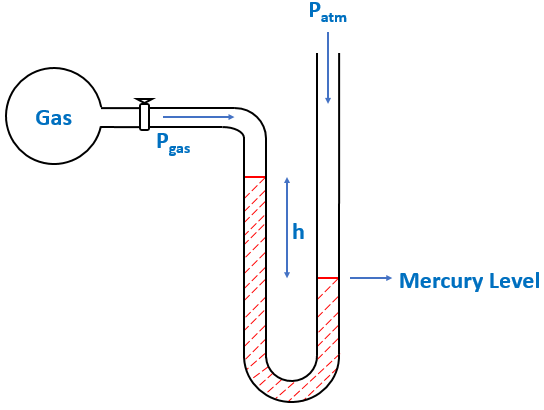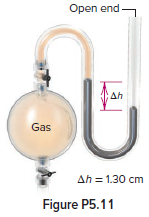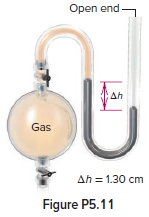# Problem: In Figure P5.11, what is the pressure of the gas in the flask (in kPa) if the barometer reads 765.2 mmHg?

###### FREE Expert Solution

When Patm is pushing harder than  Pgas$\overline{){{\mathbf{P}}}_{\mathbf{g}\mathbf{a}\mathbf{s}}{\mathbf{=}}{{\mathbf{P}}}_{\mathbf{a}\mathbf{t}\mathbf{m}}{\mathbf{-}}{\mathbf{h}}}$$\overline{){{\mathbf{P}}}_{\mathbf{g}\mathbf{a}\mathbf{s}}{\mathbf{=}}{{\mathbf{P}}}_{\mathbf{a}\mathbf{t}\mathbf{m}}{\mathbf{-}}{\mathbf{h}}}$

Given:

Patm = 765.2 mmHg
h = 1.30 cm

Convert h first from cm to mm:

87% (72 ratings)###### Problem Details

In Figure P5.11, what is the pressure of the gas in the flask (in kPa) if the barometer reads 765.2 mmHg?Frequently Asked Questions

What scientific concept do you need to know in order to solve this problem?

Our tutors have indicated that to solve this problem you will need to apply the Manometer concept. If you need more Manometer practice, you can also practice Manometer practice problems.

What professor is this problem relevant for?

Based on our data, we think this problem is relevant for Professor Waddell's class at UC.

What textbook is this problem found in?

Our data indicates that this problem or a close variation was asked in Chemistry: The Molecular Nature of Matter and Change - Silberberg 8th Edition. You can also practice Chemistry: The Molecular Nature of Matter and Change - Silberberg 8th Edition practice problems.